Example Questions

Example Question #154 : Algebra

Pets Plus makes bird houses.  Their monthly fixed expenses are $750. The cost for each bird house is$15.  The bird houses sell for $40. If Pets Plus sells 50 bird houses, what is the profit? Possible Answers: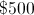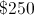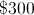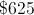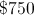Correct answer:Explanation: Let= the number of birdhouses sold each month.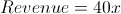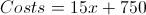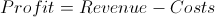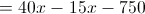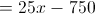Substituting in 50 forgives an answer of 500, so the profit on 50 birdhouses is$500.

Example Question #155 : Algebra

George is three times older than Joey.  The sum of their ages is 16.  What is the product of their ages?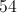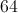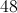Explanation:

Let= Joey's age and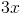= George's age.

Then the equation to solve becomes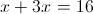.Therefore, Joey is 4 years old and George is 12 years old, so the product of their ages is 48.

Example Question #151 : Algebra

Three consecutive even numbers add to 42.  What is the middle number?Explanation:

Let= 1st even number,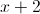= 2nd even number, and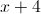= 3rd even number.

Then the equation to solve becomes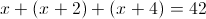.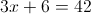Thus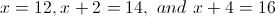, so the middle number is 14.

Example Question #157 : Algebra

Consider the following equation: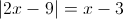Which of the following must be true?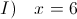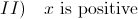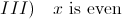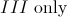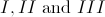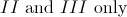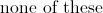Explanation:

The quantity inside the absolute value brackets must equal either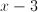or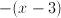, depending on whether the quantity inside the brackets is positive or negative. We therefore have two seperate equations: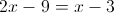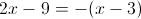To solve the first equation, add 9 to both sides:Subtractfrom both sides: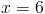This is the first solution. Now let's look at the second equation. The distributive law gives us: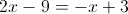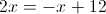Addto both sides: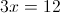Divide both sides by 3: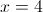Therefore,is either 4 or 6.

Statement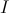does NOT have to be true becausecan also equal 4.

Statement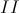must be true because both 4 and 6 are positive .

Finally, statement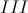always holds because 4 and 6 are both even.

Example Question #158 : Algebra

If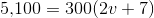,

then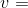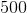Explanation:

Divide both sides by 300 to get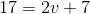.  Subtract 7 and divide by two to get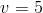.

Example Question #51 : Algebra

What is the value of (5 + x)(10 – y) when x = 3 and y = –3?

38

104

56

108

104

Explanation:

This is a simple plug-in and PEMDAS problem. First, plug in x = 3 and y = –3 into the x and y. You should follow the orders of operation and compute what is within the parentheses first and then find the product. This gives 8 * 13 = 104. The answer is 104.

Example Question #52 : Algebra

If x = 4, and y = 3x + 5, then 2y – 1 equals

33

22

47

15

33

Explanation:

Start by plugging in x = 4 to solve for y: y = 3 * 4 + 5 = 17.  Then 2 * 17 – 1 = 33

Example Question #1 : How To Find The Solution To An Equation

Sarah’s current age is three times Ron’s age two years ago. Sarah is currently 14 years older than Ron. What is the sum of Sarah and Ron’s current age?

24

36

32

34

34

Explanation:

The best way to solve this problem is to turn the two statements into equations calling Sarah’s age S and Ron’s age R. So, S = 3(R – 2) and S = 14 + R. Now substitute the value for S in the second equation for the value of S in the first equation to get 14 + R = 3(R – 2) and solve for R. So R equals 10 so S equals 24 and the sum of 10 and 24 is 34.

Example Question #54 : Algebra

A store sells potatoes for $0.24 and tomatoes for$0.76. Fred bought 12 individual vegetables. If he paid \$6.52 total, how many potatoes did Fred buy?

5

7

8

2

5

Explanation:

Set up an equation to represent the total cost in cents: 24P + 76T = 652. In order to reduce the number of variables from 2 to 1, let the # tomatoes = 12 – # of potatoes. This makes the equation 24P + 76(12 – P) = 652.

Solving for P will give the answer.

Example Question #55 : Algebra

Kim is twice as old as Claire. Nick is 3 years older than Claire. Kim is 6 years older than Emily. Their ages combined equal 81. How old is Nick?

27

22

17

13